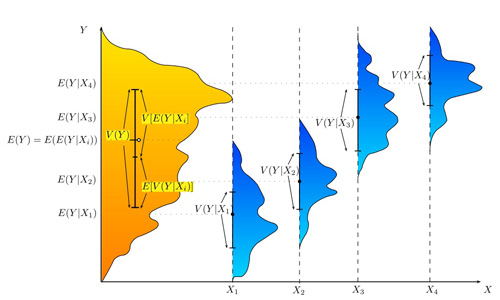#Difference Between Similar Terms and Objects

# Difference Between One way anova and two way anova## Analysis of Variances (ANOVA)

Anova refers to analysis of relationship of two groups; independent variable and dependent variable. It is basically a statistical tool that is used for testing hypothesis on the basis of experimental data. We can use anova to determine the relationship between two variables; food-habit the independent variable, and the dependent variable health condition.

The difference between one-way anova and two-way anova can be attributed to the purpose for which they are used and their concepts. The purpose of one-way anova is to see whether the data collected for one dependent variable are close to the common mean. On the other hand, two-way anova determines whether the data collected for two dependent variables converge on a common mean derived from two categories.

## One-way anova

One-way anova is used when there is only one independent variable with several groups or levels or categories, and the normally distributed response or dependent variables are measured, and the means of each group of response or outcome variables are compared.

Example of one-way anova: Consider two groups of variables, food-habit of the sample people the independent variable, with several levels as, vegetarian, non-vegetarian, and mix; and the dependent variable being number of times a person fell sick in a year. The means of response variables pertaining to each group consisting of N number of peoples are measured and compared.

## Two-way anova

When there are two independent variables each with multiple levels and one dependent variable in question the anova becomes two-way. The two-way anova shows the effect of each independent variable on the single response or outcome variables and determines whether there is any interaction effect between the independent variables. Two-way anova has been popularised by Ronald Fisher, 1925, and Frank Yates, 1934. Years later in 2005, Andrew Gelman proposed a different multilevel model approach of anova.

Example of two-way anova: If in the above example of one-way anova, we add another independent variable, ‘smoking-status’ to the existing independent variable ‘food-habit’, and multiple levels of smoking status such as non-smoker, smokers of one pack a day, and smokers of more than one pack a day, we construct a two-way anova.

## Superiority of two-way anova

Two-way anova has certain advantages over one-way anova. These are;

i. Two-way anova is more effective than one-way anova. In two-way anova there are two sources of variables or independent variables, namely food-habit and smoking-status in our example. The presence of two sources reduces the error variation, which makes the analysis more meaningful.

ii. Two-way anova helps us to assess the effects of two variables at the same time. This is not possible in one-way anova.

iii. Independence of the factors can be tested provided there are more than one observation for each factor combination or cell, and number of observations in each cell is the same. In our example factor food-habit has 3 levels and factor smoking-status has 3 levels. Thus there are 3 x 3 = 9 factor combinations or cells.

## Summary

1. Anova is a statistical analysis that is used in testing hypothesis on the basis of experimental data. Here relationships between two groups are analysed.

2. One-way anova is used when there is only one independent variable with several levels. Two-way anova is used when there are two independent variables with several levels.

3. Two-way anova is superior to one-way anova as the method has certain advantages over one-way anova.

### Search DifferenceBetween.net :

Custom Search

Help us improve. Rate this post!(2 votes, average: 3.00 out of 5)Loading...Email This Post : If you like this article or our site. Please spread the word. Share it with your friends/family.

Please note: comment moderation is enabled and may delay your comment. There is no need to resubmit your comment.

## References :

http://www.explorable.com/two-way-anova

http://www.wikipedia.org/wiki/Two-way_analysis_of_variance

http://www.math.stackexchange.com

Articles on DifferenceBetween.net are general information, and are not intended to substitute for professional advice. The information is "AS IS", "WITH ALL FAULTS". User assumes all risk of use, damage, or injury. You agree that we have no liability for any damages.

See more about : , ,